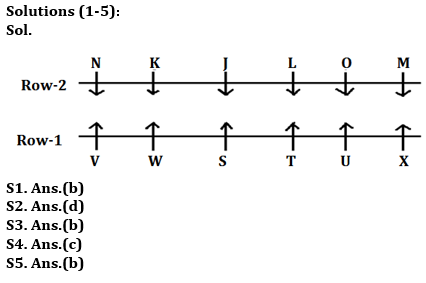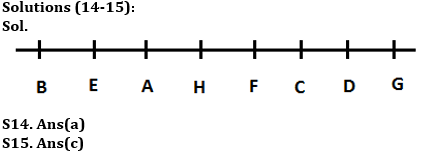Latest Banking jobs   »

# Reasoning Ability Quiz For Bank Foundation 2023 -05th May

Directions (1-5): Study the following information carefully and answer the given questions:
Twelve players are sitting in two parallel rows each containing six players in such way that they are equidistant from each other. In row 1- S, T, U, V, W and X are sitting and facing North. In row 2- J, K, L, M, N and O are sitting and facing South, but not necessarily in the same order. Each player who sits in first row faces another player of the second row.
Three players sit between U and V. The one who faces V sits third to the right of L. M faces the one who sits third to the right of S but does not face U. Immediate neighbor of K faces S. Only one player sits between J and N who faces the one who sits at the immediate left of W. Neither W nor X faces L. O does not sit at any of the extreme ends.

Q1. Who among the following sits between N and J?
(a) L
(b) K
(c) O
(d) M
(e) None of these

Q2. Who among the following faces W?
(a) J
(b) M
(c) N
(d) K
(e) O

Q3. Who among the following sits to the immediate left of O?
(a) N
(b) M
(c) J
(d) K
(e) None of these

Q4. Four of the following five belong to a group in a certain way, who among the following does not belong to that group?
(a) V
(b) N
(c) U
(d) M
(e) X

Q5. How many players sit to the right of T?
(a) Five
(b) Two
(c) One
(d) Four
(e) Three

Q6. How many pairs of letters are there in the word ‘CONFUSION’, each of which have as many letters between them in the word as they have between then in the English alphabet (both forward and backward direction)?
(a) Six
(b) Five
(c) Two
(d) Three
(e) One

Q7. Four of the following five are alike in certain way based from a group, find the one that does not belong to that group??
(a) EHL
(b) PSW
(c) ILP
(d) RUX
(e) GJN

Q8. In the given number ‘31756365842’ if ‘2’ is added to each odd number and ‘1’ is subtracted to each even number then how many digits are not repeated in the resultant thus obtained?
(a) one
(b) two
(c) three
(d) four
(e) None

Directions (9-12): Study the following information carefully and answer the given questions.
Amongst Six friends M, N, O, P, Q and R, each got different marks in the examination. P score just more than R. Not more than three persons score more marks than O. M score more than Q and less than R. P does not score maximum marks. The one who score least marks score 31 marks. Q score is not odd number. The one who score 4th highest score 52 marks.

Q9. Who scored the highest marks?
(a) N
(b) R
(c) Q
(d) M
(e) O

Q10. Who among the following Person has scored third highest marks?
(a) Q
(b) R
(c) N
(d) Q
(e) Either (b) or (c)

Q11. If P’s score 61 marks than what may be the score of R?
(a) 58
(b) 68
(c) 51
(d) 64
(e) None of these

Q12. Who among the following person score just more than M?
(a) O
(b) P
(c) N
(d) Q
(e) None of these

Q13. Kamal is 15th from the left end of a row of 45 students and Preet is 28th from the right end in the same row. How many students are there between them in the row?
(a) 3
(b) 2
(c) 4
(d) 1
(e) None of these

Directions (14-15): Study the following information carefully and answer the given questions.
Eight persons are sitting in a row facing south but not necessarily in the same order. H sits second to the right of C and second to the left of E. B is not an immediate neighbour of H and C. F is second to the right of D. G is not the neighbour of E. Only two persons sit to the right of A. G is not an immediate neighbour of A and doesn’t sit at extreme right end.

Q14. Who among the following is an immediate neighbour of both H and E?
(a) A
(b) B
(c) C
(d) G
(e) None of these

Q15. In which of the following pairs the second person is sitting on the right of the first person?
(a) A, H
(b) C, D
(c) G, H
(d) E, H
(e) F, D

SolutionsS6. Ans.(a)

S7. Ans. (d)

S8. Ans.(b)
Sol. ‘31756365842’ changes to ‘53975557731’ two numbers i.e. 9 and 1 are not repeated.

Solutions (9-12):
Sol. O > P > R > M (52) > Q > N (31)

S9. Ans(e)
S10. Ans(b)
S11. Ans(a)
S12. Ans(e)

S13. Ans(b)
Sol. Kamal position from right end = (45+1-15) = 31
Students between them = (31-28-1) = 2## FAQs

### What is the selection process of the Bank Clerk?

The selection process of the Bank Clerk is Prelims & Mains.

#### Congratulations!Union Budget 2023-24: Free PDF